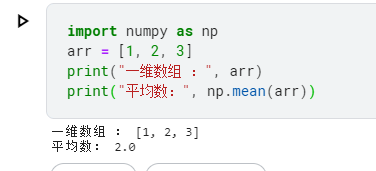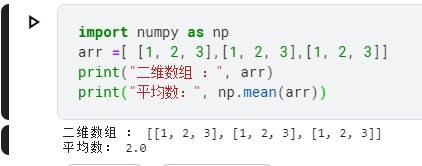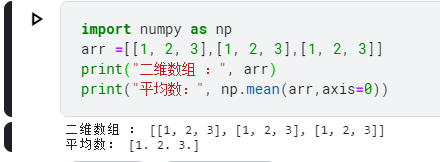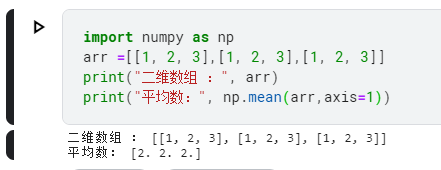# numpy.mean()的使用

arr表示输入的数据，可以是一维数组或二维数组
axis表示计算平均数的轴，
dtype表示计算平均数时使用的数据类型。默认为float64

## 示范

``````// 一维数组
import numpy as np
arr = [1, 2, 3]
print("一维数组 ：", arr)
print("平均数：", np.mean(arr))

``````
• 1
• 2
• 3
• 4
• 5
• 6``````// 二维数组求所有数的平均数
import numpy as np
arr =[ [1, 2, 3],[1, 2, 3],[1, 2, 3]]
print("二维数组 ：", arr)
print("平均数：", np.mean(arr))
``````
• 1
• 2
• 3
• 4
• 5``````// 二维数组按列求平均数
import numpy as np
arr =[[1, 2, 3],[1, 2, 3],[1, 2, 3]]
print("二维数组 ：", arr)
print("平均数：", np.mean(arr,axis=0))
``````
• 1
• 2
• 3
• 4
• 5``````// 二维数组按行求平均数
import numpy as np
import numpy as np
arr =[[1, 2, 3],[1, 2, 3],[1, 2, 3]]
print("二维数组 ：", arr)
print("平均数：", np.mean(arr,axis=1))
``````
• 1
• 2
• 3
• 4
• 5
• 6dtype参数不常用，如果设置成float32，则结果的分辨率较低。